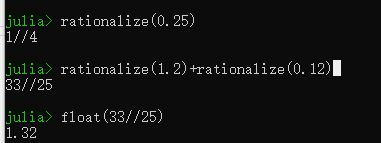# 在Julia里计算“1.2+0.21”的结果有问题啊

julia> 1.2 + 0.12

1.3199999999999998

1 个赞

Julia的浮点数运算是支持IEEE754标准的，因此浮点数的代数运算不满足结合律:

``````julia> 0.1 + 0.2 + 0.3
0.6000000000000001

julia> 0.1 + (0.2 + 0.3)
0.6
``````

``````0.1 + 0.2 + 0.3 == 0.1 + (0.2 + 0.3)
false

0.1 + 0.2 + 0.3 ≈ 0.1 + (0.2 + 0.3)
true
``````

2 个赞

``````help?> ≈
"≈" can be typed by \approx<tab>

search: ≈

isapprox(x, y; rtol::Real=atol>0 ? 0 : √eps, atol::Real=0, nans::Bool=false, norm::Function)

Inexact equality comparison: true if norm(x-y) <= max(atol, rtol*max(norm(x), norm(y))). The default atol is zero and the default rtol
depends on the types of x and y. The keyword argument nans determines whether or not NaN values are considered equal (defaults to false).
``````

You can also get information on how to type a symbol by entering it in the REPL help, i.e. by typing `?` and then entering the symbol in the REPL (e.g., by copy-paste from somewhere you saw the symbol).

1 个赞

1 个赞
``````julia> exp(im*pi) ≈ -1
true

julia> exp(im*pi) + 1 ≈ 0
false
``````

``````julia> eps(1.)
2.220446049250313e-16

julia> eps(0.)
5.0e-324
``````
1 个赞

rationalize(0.25)
julia是支持分数的，分数是没有误差，当然，开方，无理数进入运算，又会隐式转换成浮点了